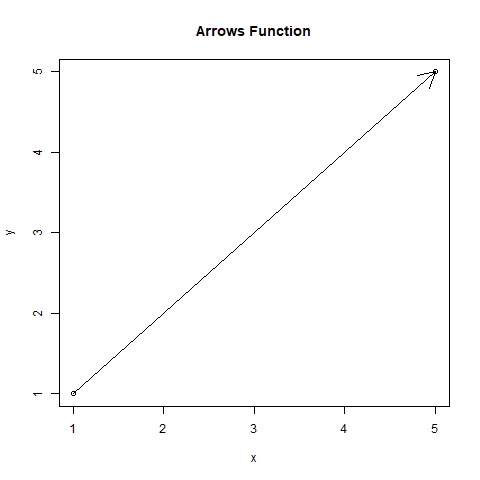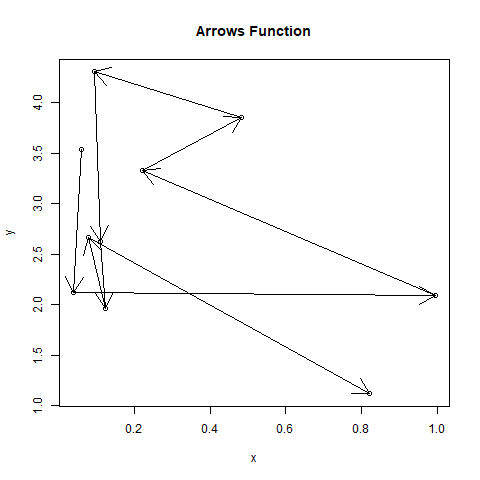# Plot Arrows Between Points in a Graph in R Programming – arrows() Function

`arrows()` function in R Language is used to create arrows between the points on the graph specified.

Syntax: arrows(x0, y0, x1, y1, length)

Parameters:
x0: represents x-coordinate of point from which to draw the arrow
y0: represents y-coordinate of point from which to draw the arrow
x1: represents x-coordinate of point to which the arrow is drawn
y1: represents y-coordinate of point to which the arrow is drawn
length: represents length of the edge of the arrow head (in inches)

Example 1:

 `# Specifying points ` `x0 <- 1 ` `y0 <- 1 ` `x1 <- 5 ` `y1 <- 5 ` `x <- ``c``(x0, x1) ` `y <- ``c``(y0, y1) ` ` `  `# Output to be present as PNG file ` `png``(file = ``"arrows1GFG.png"``) ` ` `  `# Create plot graph ` `plot``(x, y, main = ``"Arrows Function"``) ` ` `  `# Create arrow between the points ` `arrows``(x0, y0, x1, y1) ` ` `  `# Saving the file ` `dev.off``() `

Output:Example 2:

 `# Specifying points ` `x <- ``runif``(10, 0, 1) ` `y <- ``runif``(10, 1, 5) ` ` `  `# Output to be present as PNG file ` `png``(file = ``"arrows2GFG.png"``) ` ` `  `# Create plot graph ` `plot``(x, y, main = ``"Arrows Function"``) ` ` `  `# Create arrow between the points ` `s <- ``seq``(``length``(x) - 1) ` `arrows``(x[s], y[s], x[s + 1], y[s + 1]) ` ` `  `# Saving the file ` `dev.off``() `

Output:My Personal Notes arrow_drop_upBlockchain Enthusiast

If you like GeeksforGeeks and would like to contribute, you can also write an article using contribute.geeksforgeeks.org or mail your article to contribute@geeksforgeeks.org. See your article appearing on the GeeksforGeeks main page and help other Geeks.

Please Improve this article if you find anything incorrect by clicking on the "Improve Article" button below.

Article Tags :

Be the First to upvote.

Please write to us at contribute@geeksforgeeks.org to report any issue with the above content.